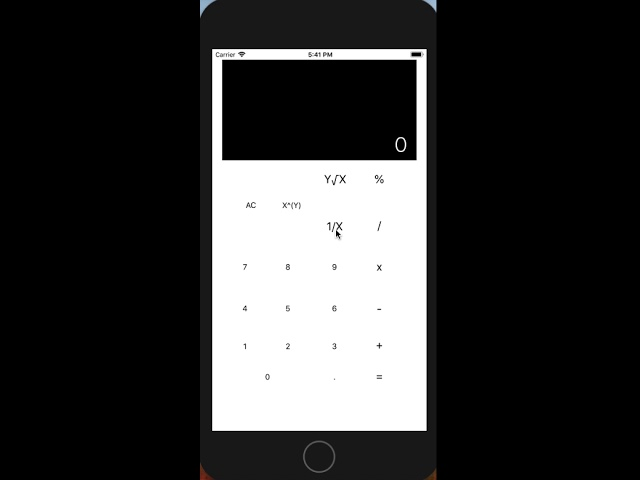Play Video

## Calculator

The calculator is a simple but necessary mathematical tool for everyone. The right calculation APP can take a headache out of dealing with complex calculations. On this APP, you can add, subtract, multiply and divide numbers. Also, it can calculate fraction and find the result of the square root. Let's have fun with learning math!

Wenzi Suo

2018 Summer Camp Class 4

iOS coding development student